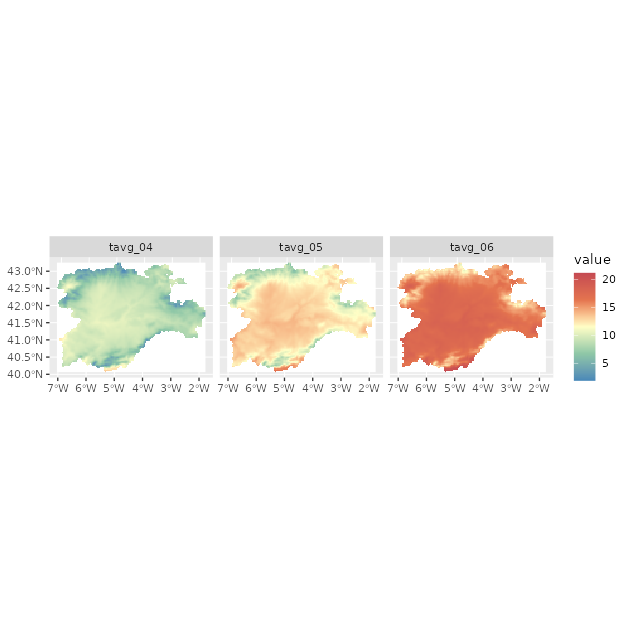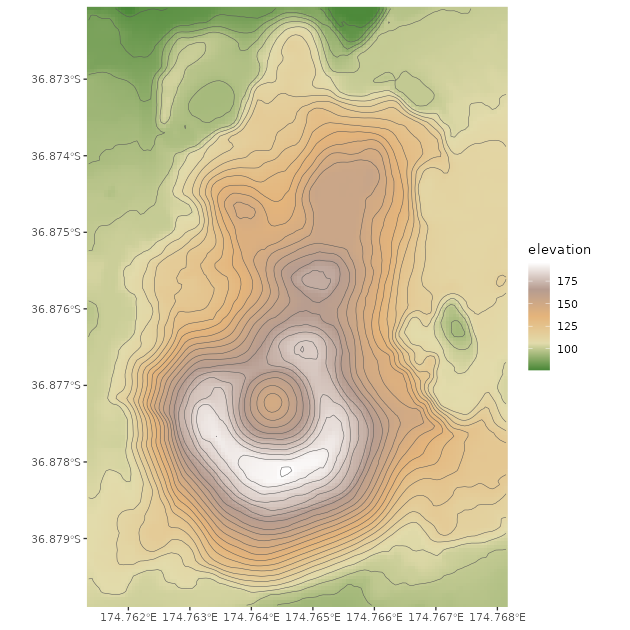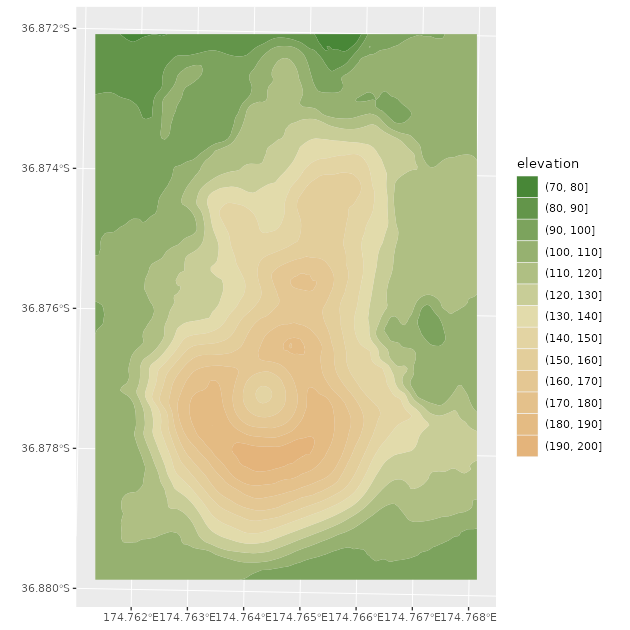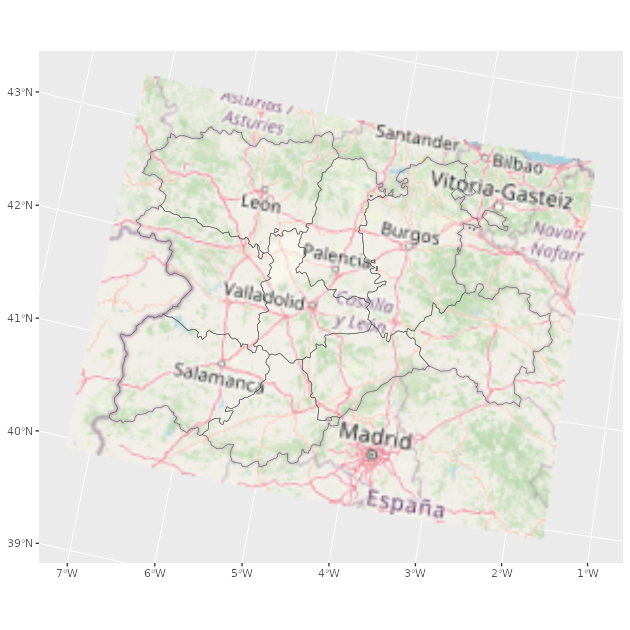# Welcome to tidyterra

## Welcome to {tidyterra}

tidyterra is a package that add common methods from the tidyverse for SpatRaster and SpatVectors objects created with the {terra} package. It also adds specific `geom_spat*()` functions for plotting these kind of objects with {ggplot2}.

### Why {tidyterra}?

Spat* objects are not like regular data frames. They are a different type of objects, implemented via the S4 object system, and have their own syntax and computation methods, implemented on the {terra} package.

By implementing tidyverse methods for these objects, and more specifically {dplyr} and {tidyr} methods, a useR can now work more easily with Spat*, just like (s)he would do with tabular data.

Note that in terms of performance, {terra} is much more optimized for working for this kind of objects, so it is recommended also to learn a bit of {terra} syntax. Each function of {tidyterra} refers (when possible) to the corresponding equivalent on {terra}.

## A note for advanced {terra} users

As previously mentioned, {tidyterra} is not optimized in terms of performance. Specially when working with `filter()` and `mutate()` methods, it can be slow.

As a rule of thumb, {tidyterra} can handle objects with less than 10.000.000 slots of information(i.e., `terra::ncell(a_rast) * terra::nlyr(a_rast) < 10e6`).

## Get started with {tidyterra}

``````library(tidyterra)
#>
#> Attaching package: 'tidyterra'
#> The following object is masked from 'package:stats':
#>
#>     filter
library(dplyr)
#>
#> Attaching package: 'dplyr'
#> The following objects are masked from 'package:stats':
#>
#>     filter, lag
#> The following objects are masked from 'package:base':
#>
#>     intersect, setdiff, setequal, union
library(tidyr)``````

Currently, the following methods are available:

tidyverse method SpatVector SpatRaster
`tibble::as_tibble()` ✔️ ✔️
`dplyr::select()` ✔️ ✔️ Select layers
`dplyr::mutate()` ✔️ ✔️ Create /modify layers
`dplyr::transmute()` ✔️ ✔️
`dplyr::filter()` ✔️ ✔️ Modify cells values and (additionally) remove outer cells.
`dplyr::slice()` ✔️ ✔️ Additional methods for slicing by row and column.
`dplyr::pull()` ✔️ ✔️
`dplyr::rename()` ✔️ ✔️
`dplyr::relocate()` ✔️ ✔️
`dplyr::distinct()` ✔️
`dplyr::arrange()` ✔️
`dplyr::glimpse()` ✔️ ✔️
`dplyr::inner_join()` family ✔️
`dplyr::summarise()` ✔️
`dplyr::group_by()` family ✔️
`dplyr::rowwise()` ✔️
`dplyr::count()`, `tally()` ✔️
`dplyr::bind_cols()` / `dplyr::bind_rows()` ✔️ as `bind_spat_cols()` / `bind_spat_rows()`
`tidyr::drop_na()` ✔️ ✔️ Remove cell values with `NA` on any layer. Additionally, outer cells with `NA` are removed.
`tidyr::replace_na()` ✔️ ✔️
`ggplot2::autoplot()` ✔️ ✔️
`ggplot2::fortify()` ✔️ to sf via `sf::st_as_sf()` To a tibble with coordinates.
`ggplot2::geom_*()` ✔️ `geom_spatvector()` ✔️ `geom_spatraster()` and `geom_spatraster_rgb()`.

Let’s see some of them in action:

### SpatRasters

See an example with SpatRaster objects:

``````library(terra)
f <- system.file("extdata/cyl_temp.tif", package = "tidyterra")

temp <- rast(f)

temp
#> class       : SpatRaster
#> dimensions  : 87, 118, 3  (nrow, ncol, nlyr)
#> resolution  : 3881.255, 3881.255  (x, y)
#> extent      : -612335.4, -154347.3, 4283018, 4620687  (xmin, xmax, ymin, ymax)
#> coord. ref. : World_Robinson
#> source      : cyl_temp.tif
#> names       :   tavg_04,   tavg_05,  tavg_06
#> min values  :  1.885463,  5.817587, 10.46338
#> max values  : 13.283829, 16.740898, 21.11378

mod <- temp %>%
select(-1) %>%
mutate(newcol = tavg_06 - tavg_05) %>%
relocate(newcol, .before = 1) %>%
replace_na(list(newcol = 3)) %>%
rename(difference = newcol)

mod
#> class       : SpatRaster
#> dimensions  : 87, 118, 3  (nrow, ncol, nlyr)
#> resolution  : 3881.255, 3881.255  (x, y)
#> extent      : -612335.4, -154347.3, 4283018, 4620687  (xmin, xmax, ymin, ymax)
#> coord. ref. : World_Robinson
#> source(s)   : memory
#> names       : difference,   tavg_05,  tavg_06
#> min values  :   2.817647,  5.817587, 10.46338
#> max values  :   5.307511, 16.740898, 21.11378``````

On the previous example, we had:

• Eliminated the first layer of the raster `tavg_04`.

• Created a new layer `newcol` as the difference of the layers `tavg_05` and `tavg_06.`

• Relocated `newcol`as the first layer of the SpatRaster

• Replaced the `NA` cells on `newcol` with `3`.

• Renamed `newcol` to difference.

In all the process, the essential properties of the SpatRaster (number of cells, columns and rows, extent, resolution and coordinate reference system) have not been modified. Other methods as `filter()`, `slice()` or `drop_na()` can modify these properties, as they would do when applied to a data frame (number of rows would be modified on that case).

### SpatVectors

`tidyterra >= 0.4.0` provides support to SpatVectors for most of the {dplyr} methods, so it is possible to arrange, group and summarise information of SpatVectors

``````lux <- system.file("ex/lux.shp", package = "terra")

v_lux <- vect(lux)

v_lux %>%
# Create categories
mutate(gr = cut(POP / 1000, 5)) %>%
group_by(gr) %>%
# Summary
summarise(
n = n(),
tot_pop = sum(POP),
mean_area = mean(AREA)
) %>%
# Arrange
arrange(desc(gr))
#>  class       : SpatVector
#>  geometry    : polygons
#>  dimensions  : 3, 4  (geometries, attributes)
#>  extent      : 5.74414, 6.528252, 49.44781, 50.18162  (xmin, xmax, ymin, ymax)
#>  coord. ref. : lon/lat WGS 84 (EPSG:4326)
#>  names       :          gr     n tot_pop mean_area
#>  type        :      <fact> <int>   <int>     <num>
#>  values      :   (147,183]     2  359427       244
#>                (40.7,76.1]     1   48187       185
#>                (4.99,40.7]     9  194391     209.8``````

As in the case of SpatRaster, basic properties as the geometry and the CRS are preserved.

## Plotting with {ggplot2}

### SpatRasters

{tidyterra} provides several `geom_*` for SpatRasters. When the SpatRaster has the CRS informed (i.e. `terra::crs(a_rast) != ""`), the geom uses `ggplot2::coord_sf()`, and may be also reprojected for adjusting the coordinates to other spatial layers:

``````library(ggplot2)

# A faceted SpatRaster

ggplot() +
geom_spatraster(data = temp) +
facet_wrap(~lyr) +
scale_fill_whitebox_c(
palette = "muted",
na.value = "white"
)````````````

# Contour lines for a specific layer

f_volcano <- system.file("extdata/volcano2.tif", package = "tidyterra")
volcano2 <- rast(f_volcano)

ggplot() +
geom_spatraster(data = volcano2) +
geom_spatraster_contour(data = volcano2, breaks = seq(80, 200, 5)) +
scale_fill_whitebox_c() +
coord_sf(expand = FALSE) +
labs(fill = "elevation")````````````

# Contour filled

ggplot() +
geom_spatraster_contour_filled(data = volcano2) +
scale_fill_whitebox_d(palette = "atlas") +
labs(fill = "elevation")``````With {tidyterra} you can also plot RGB SpatRasters to add imagery to your plots:

``````# Read a vector

f_v <- system.file("extdata/cyl.gpkg", package = "tidyterra")
v <- vect(f_v)

f_rgb <- system.file("extdata/cyl_tile.tif", package = "tidyterra")

r_rgb <- rast(f_rgb)

rgb_plot <- ggplot(v) +
geom_spatraster_rgb(data = r_rgb) +
geom_spatvector(fill = NA, size = 1)

rgb_plot````````````# Change CRS automatically

rgb_plot +
coord_sf(crs = 3857)``````